$$\require{cancel}$$

# B12: Kirchhoff’s Rules, Terminal Voltage

There are two circuit-analysis laws that are so simple that you may consider them “statements of the obvious” and yet so powerful as to facilitate the analysis of circuits of great complexity. The laws are known as Kirchhoff’s Laws. The first one, known both as “Kirchhoff’s Voltage Law” and “The Loop Rule” states that, starting on a conductor, if you drag the tip of your finger around any loop in the circuit back to the original conductor, the sum of the voltage changes experienced by your fingertip will be zero. (To avoid electrocution, please think of the finger dragging in an actual circuit as a thought experiment.)

# Kirchhoff’s Voltage Law (a.k.a. the Loop Rule)

To convey the idea behind Kirchhoff’s Voltage Law, I provide an analogy. Imagine that you are exploring a six-story mansion that has $$20$$ staircases. Suppose that you start out on the first floor. As you wander around the mansion, you sometimes go up stairs and sometimes go down stairs. Each time you go up stairs, you experience a positive change in your elevation. Each time you go down stairs, you experience a negative change in your elevation. No matter how convoluted the path of your explorations might be, if you again find yourself on the first floor of the mansion, you can rest assured that the algebraic sum of all your elevation changes is zero.

To relate the analogy to a circuit, it is best to view the circuit as a bunch of conductors connected by circuit elements (rather than the other way around as we usually view a circuit). Each conductor in the circuit is at a different value of electric potential (just as each floor in the mansion is at a different value of elevation). You start with your fingertip on a particular conductor in the circuit, analogous to starting on a particular floor of the mansion. The conductor is at a particular potential. You probably don’t know the value of that potential any more than you know the elevation that the first floor of the mansion is above sea level. You don’t need that information. Now, as you drag your finger around the loop, as long as you stay on the same conductor, your fingertip will stay at the same potential. But, as you drag your fingertip from that conductor, through a circuit element, to the next conductor on your path, the potential of your fingertip will change by an amount equal to the voltage across the circuit element (the potential difference between the two conductors). This is analogous to climbing or descending a flight of stairs and experiencing a change in elevation equal to the elevation difference between the two floors.

If you drag your fingertip around the circuit in a loop, back to the original conductor, your finger is again at the potential of that conductor. As such, the sum of the changes in electric potential experienced by your finger on its traversal of the loop must be zero. This is analogous to stating that if you start on one floor of the mansion, and, after wandering through the mansion, up and down staircases, you end up on the same floor of the mansion, your total elevation change is zero. In dragging your finger around a closed loop of a circuit (in any direction you want, regardless of the current direction) and adding each of the voltage changes to a running total, the critical issue is the algebraic sign of each voltage change. In the following example we show the steps that you need to take to get those signs right, and to prove to the reader of your solution that they are correct.

Example

Find the current through each of the resistors in the following circuit.Before we get started, let’s define some names for the given quantities:Each two-terminal circuit element has one terminal that is at a higher potential than the other terminal. The next thing we want to do is to label each higher potential terminal with a “$$+$$” and each lower-potential terminal with a “$$-$$”. We start with the seats of EMF. They are trivial. By definition, the longer parallel line segment, in the symbol used to depict a seat of EMF, is at the higher potential.Next we define a current variable for each “leg” of the circuit. A “leg” of the circuit extends from a point in the circuit where three or more wires are joined (called a junction) to the next junction. All the circuit elements in any one leg of the circuit are in series with each other, so, they all have the same current through them.Note

In defining your current variables, the direction in which you draw the arrow in a particular leg of the circuit, is just a guess. Don’t spend a lot of time on your guess. It doesn’t matter. If the current is actually in the direction opposite that in which your arrow points, you will simply get a negative value for the current variable. The reader of your solution is responsible for looking at your diagram to see how you have defined the current direction and for interpreting the algebraic sign of the current value accordingly.

Now, by definition, the current is the direction in which positive charge carriers are flowing. The charge carriers lose electric potential energy when they go through a resistor, so, they go from a higher-potential conductor, to a lower-potential conductor when they go through a resistor. That means that the end of the resistor at which the current enters the resistor is the higher potential terminal ($$+$$), and, the end at which the current exits the resistor is the lowerpotential terminal ($$-$$) of the resistor.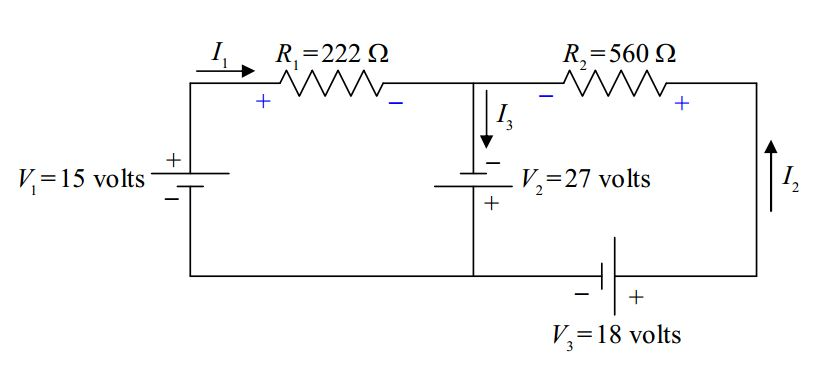Now let’s define some variable names for the resistor voltages:Note that the $$+$$ and $$–$$ signs on the resistors are important parts of our definitions of $$V_{R1}$$ and $$V_{R2}$$. If, for instance, we calculate $$V_{R1}$$ to have a positive value, then, that means that the left (as we view it) end of $$V_{R1}$$ is at a higher potential than the right end (as indicated in our diagram). If $$V_{R1}$$ turns out to be negative, that means that the left end of $$R_1$$ is actually at a lower potential than the right end. We do not have to do any more work if $$V_{R1}$$ turns out to be negative. It is incumbent upon the reader of our solution to look at our circuit diagram to see what the algebraic sign of our value for $$V_{R1}$$ means.

With all the circuit-element terminals labeled “$$+$$” for “higher potential” or “$$–$$” for “lower potential,” we are now ready to apply the Loop Rule. I’m going to draw two loops with arrowheads. The loop that one draws is not supposed to be a vague indicator of direction but a specific statement that says, “Start at this point in the circuit. Go around this loop in this direction, and, end at this point in the circuit.” Also, the starting point and the ending point should be the same. In particular, they must be on the same conductor. (Never start the loop on a circuit element.) In the following diagram are the two loops, one labeled 1 and the other labeled 2.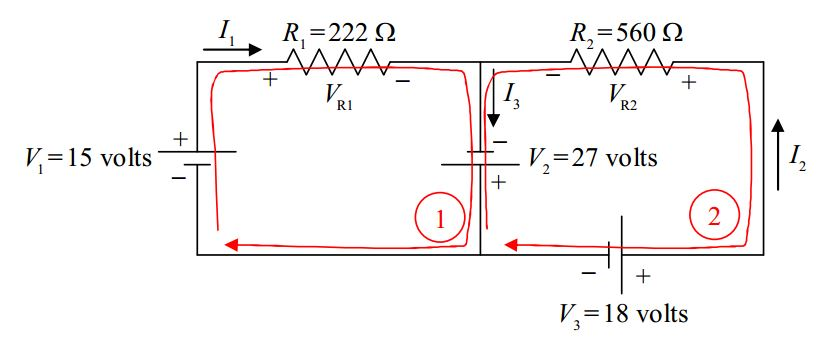Now we write KVL 1 to tell the reader that we are applying the Loop Rule (Kirchhoff’s Voltage Law) using loop 1, and transcribe the loop equation from the circuit diagram:

$KVL1+V_1-V_{R1}+V_2=0$

The equation is obtained by dragging your fingertip around the exact loop indicated and recording the voltage changes experienced by your fingertip, and then, remembering to write “$$= 0$$.” Starting at the point on the circuit closest to the tail of the loop 1 arrow, as we drag our finger around the loop, we first traverse the seat of EMF, $$V_1$$. In traversing $$V_1$$ we go from lower potential ($$-$$) to higher potential ($$+$$). That means that the finger experiences a positive change in potential, hence, $$V_1$$ enters the equation with a positive sign. Next we come to resistor $$R_1$$. In traversing $$R_1$$ we go from higher potential ($$+$$) to lower potential ($$-$$). That’s a negative change in potential. Hence, $$V_{R1}$$ enters our loop equation with a negative sign. As we continue our way about the loop we come to the seat of EMF $$V_2$$ and go from lower potential ($$-$$) to higher potential ($$+$$) as we traverse it. Thus, $$V_2$$ enters the loop equation with a positive sign. Finally, we arrive back at the starting point. That means that it is time to write “$$= 0$$.”

We transcribe the second loop equation in the same fashion:

$KVL2-V_2+V_{R2}-V_3=0$

With these two equations in hand, and knowing that $$V_{R1} = I_1R_1$$ and $$V_{R2} = I_2 R_2$$, the solution to the example problem is straightforward. (We leave it as an exercise for the reader.) It is now time to move on to Kirchhoff’s other law.

# Kirchhoff’s Current Law (a.k.a. the Junction Rule)

Kirchhoff’s junction rule is a simple statement of the fact that charge does not pile up at a junction. (Recall that a junction is a point in a circuit where three or more wires are joined together.) I’m going to state it two ways and ask you to pick the one you prefer and use that one. One way of stating it is to say that the net current into a junction is zero. Check out the circuit from the example problem: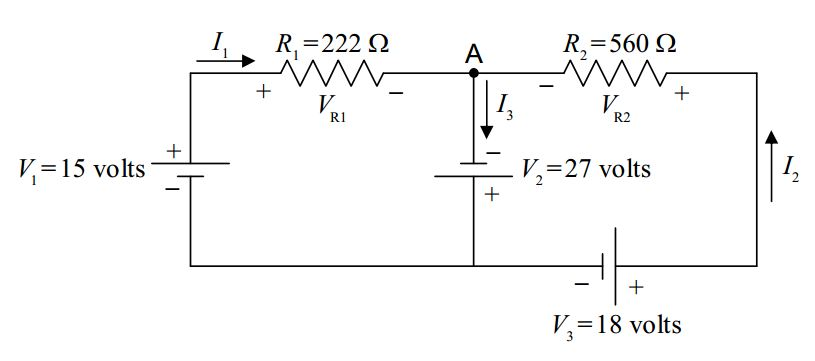In this copy of the diagram of that circuit, I put a dot at the junction at which I wish to apply Kirchhoff’s Current Law, and, I labeled that junction “$$A$$.”

Note that there are three legs of the circuit attached to junction $$A$$. In one of them, current $$I_1$$ flows toward the junction. In another, current $$I_2$$ flows toward the junction. In the third leg, current $$I_3$$ flows away from the junction. A current away from the junction counts as the negative of that value of current, toward the junction. So, applying Kirchhoff’s Current Law in the form, “The net current into any junction is zero,” to junction $$A$$ yields:

$\frac{\mbox{KCL A}}{I_1}+I_2-I_3=0$

Note the negative sign in front of $$I_3$$. A current of $$-I_3$$ into junction $$A$$ is the same thing as a current of $$I_3$$ out of that junction, which is exactly what we have.

The other way of stating Kirchhoff’s Current Law is, “The current into a junction is equal to the current out of that junction.” In this form, in applying Kirchhoff’s Current Law to junction $$A$$ in the circuit above, one would write:

$\frac{\mbox{KCL A}}{I_1}+I_2=I_3$

Obviously, the two results are the same.

# Terminal Voltage – A More Realistic Model for a Battery or DC Electrical Power Source

Our model for a battery up to this point has been a seat of EMF. I said that a seat of EMF can be considered to be an ideal battery. This model for a battery is good as long as the battery is fairly new and unused and the current through it is small. Small compared to what? How small? Well, small enough so that the voltage across the battery when it is in the circuit is about the same as it is when it is not in any circuit. How close to being the same? That depends on how accurate you want your results to be. The voltage across a battery decreases when you connect the battery in a circuit. If it decreases by five percent and you calculate values based on the voltage across the battery when it is in no circuit, your results will probably be about $$5%$$ off.

A better model for a battery is an ideal seat of EMF in series with a resistor. A battery behaves very much as if it consisted of a seat of EMF in series with a resistor, but, you can never separate the seat of EMF from the resistor, and if you open up a battery you will never find a resistor in there. Think of a battery as a black box containing a seat of EMF and a resistor. The resistor in this model is called the internal resistance of the battery.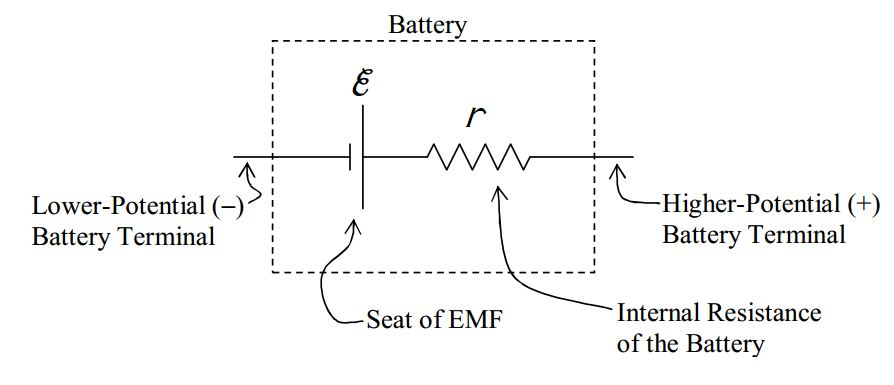The point at which the seat of EMF is connected to the internal resistance of the battery is inaccessible. The potential difference between the terminals of the battery is called the terminal voltage of the battery. When the battery is not part of a circuit, the terminal voltage is equal to the EMF. You can deduce this from the fact that when the battery is not part of a circuit, there can be no current through the resistor. If there is no current through the resistor than the two terminals of the resistor must be at one and the same value of electric potential. Thus, in the diagram above, the right end of the resistor is at the same potential as the high-potential terminal of the seat of EMF.

Now, let’s put the battery in a circuit: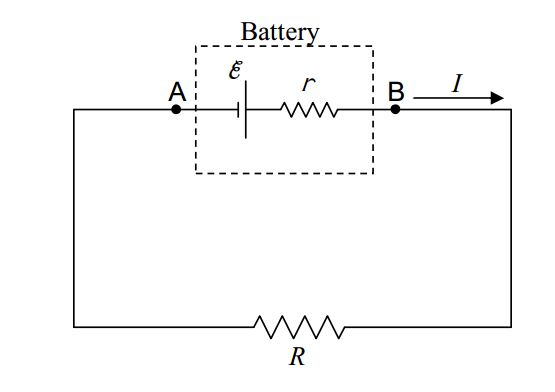I’ve indicated the two points $$A$$ and $$B$$ on the circuit for communication purposes. The terminal voltage is the voltage from $$A$$ to $$B$$ ($$V_{AB}$$). If you trace the circuit, with your fingertip, from $$A$$ to $$B$$, the terminal voltage (how much higher the potential is at $$B$$ than it is at $$A$$) is just the sum of the voltage changes your finger experiences along the path. (Note that this time, we are not going all the way around a loop. We do not wind up on the same conductor upon which we started. So, the sum of the voltage changes from $$A$$ to $$B$$ is not zero.) To sum the voltage changes from $$A$$ to $$B$$, I will mark the terminals of the components between $$A$$ and $$B$$ with “$$+$$” for higher potential and “$$-$$” for lower potential.

First the seat of EMF: That’s trivial. The shorter side of the EMF symbol is the lower potential ($$-$$) side and the longer side is the higher potential ($$+$$) side.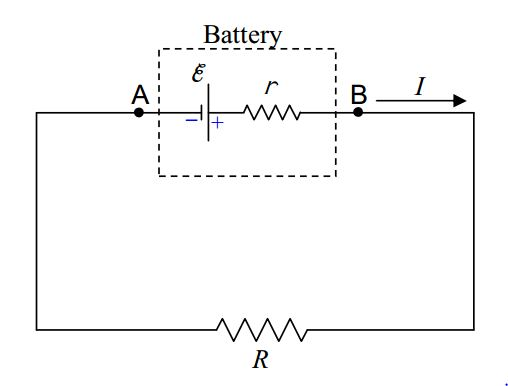Now, for the internal resistance of the battery: The end of the internal resistance r that the current enters is the higher-potential ($$+$$) end, and, the end that it exits is the lower potential ($$-$$) end.Note that I have also defined, in the preceding diagram, the variable $$V_r$$ for the voltage across the internal resistance of the battery. Remember, to get the terminal voltage $$V_{AB}$$ of the battery, all we have to do is to sum the potential changes that our fingertip would experience if we were to drag it from $$A$$ to $$B$$ in the circuit. (This is definitely a thought experiment because we can’t get our fingertip inside the battery.)

$V_{AB}=\varepsilon-V_{r}$

$V_{AB}=\varepsilon-Ir$

Note that, in the second line, I used the definition of resistance ($$V=IR$$) in the form $$V_r = Ir$$, to replace $$V_r$$ with $$Ir$$.

We have been consistent, in this book, with the convention that a double subscript such as $$AB$$ can be read “$$A$$ to $$B$$” meaning, in the case at hand, that $$V_{AB}$$ is the sum of the potential changes from $$A$$ to $$B$$ (rather than the other way around), in other words, that $$V_{AB}$$ is how much higher the electric potential at point $$B$$ is than the electric potential at point $$A$$. Still, there are some books out there that take $$V_{AB}$$ (all by itself) to mean the voltage of $$A$$ with respect to $$B$$ (which is the negative of what we mean by it). So, for folks that may have used a different convention than you use, it is a good idea to diagrammatically define exactly what you mean by $$V_{AB}$$. Putting a voltmeter, labeled to indicate that it reads $$V_{AB}$$, and labeled to indicate which terminal is its “$$+$$” terminal and which is its “$$-$$” terminal is a good way to do this.